Translations and Reflections

Translations

Given an equation in x and y, the following changes in the equation result in the indicated changes in the graph (where):

 Replacing Translation x by x-h h units to the right x by x+h h units to the left y by y-h h units up y by y+h h units down

Reflections

Given an equation in x and y, the following changes in the equation result in the indicated changes in the graph:

 Replacing Reflection x by -x in the y-axis y by -y in the x-axis x by -x and y by -y in the origin x by y and y by x in the line y=x

Ex 1 Find an equation of the curve which results by translating the graph of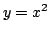5 units to the right and 3 units down.

Sol Replacingbyandbygives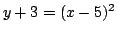or.

Ex 2 Explain how to obtain the graph offrom the graph ofusing translations and reflections.

Sol a First shift the graph of2 units to the left to get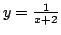, and then reflect in the y-axis to get.

Sol b First reflect the graph in the y-axis to get, and then shift the graph 2 units to the right to get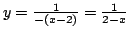.

Pr A Find an equation of the graph whic is obtained by reflecting the graph ofin the line.

Pr B Find an equation of the graph which results from reflecting the graph ofin the x-axis, and then shifting the resulting graph 3 units up.

Pr C Find an equation of the graph which is obtained by reflecting the graph ofin the y-axis, and then shifting the resulting graph 4 units to the right.

Pr 1 Find an equation of the graph which results from translating the graph of5 units to the left and 4 units up.

Pr 2 Find an equation of the graph which results from reflecting the graph ofin the y-axis, and then shifting the graph 3 units to the left.

Pr 3 Find an equation of the graph which results from shifting the graph of3 units to the left, and then reflecting in the y-axis.

Pr 4 Explain how to obtain the graph of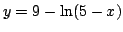from the graph ofusing translations and reflections.

Pr 5 Find an equation of the graph which is obtained by reflecting the graph ofin the line, and then shifting the resulting graph 4 units down.

Pr 6 Find an equation of the graph which is obtained by shifting the graph of4 units down, and then reflecting the resulting graph in the line.

Pr 7 Find an equation of the graph which is obtained by reflecting the graph ofin the line, then shifting the resulting graph 3 units to the right, and then shifting the graph which results 4 units up.

Pr 8 Explain how to obtain the graph of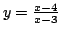from the graph ofusing translations and reflections.